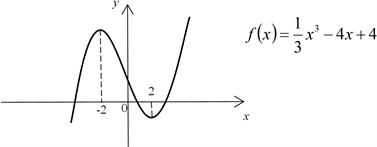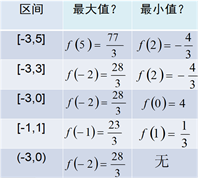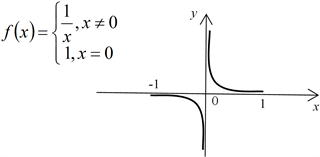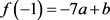﻿ 数学新授课课堂教学研究——“函数的最大(小)值与导数”的教学与感悟

# 数学新授课课堂教学研究——“函数的最大(小)值与导数”的教学与感悟A Study of Classroom Teaching of New Mathematic Lessons—Teaching and Inspiration of “Maximum (Minimum) Value and Derivative of Function”

Abstract: This paper describes the teaching process of the first class hour of “Maximum (Minimum) Value and Derivative of Function” in detail. During this process, under the guidance of heuristic teaching principles, students are naturally introduced to enter the learning of new knowledge by means of question-and-answer, which motivates their interest in learning and enables them to actively participate in the lesson. Some pieces of inspiration can be obtained from the teaching of this lesson: the importance of natural guidance in teaching new mathematic lessons; the significance of students-oriented classroom teaching mode; the mathematics teaching concept that practice is much better than just viewing; the necessity of knowledge summary. Finally, this paper analyzes and summarizes the shortcomings and expectations of this lesson.

1. 基本情况

1.1. 授课对象

1.2. 教材分析

“函数的最大(小)值与导数”是《普通高中课程标准试验教科书·数学(选修2-2)》第一章“导数及其应用”中的内容。在社会生活实践中，为了发挥最大的经济效益，常常遇到如何能使用料最省、产量最高、效益最大等问题，这些问题的解决常常可转化为求一个函数的最大值和最小值问题，而导数是求函数最大(小)值的有力工具。本节课是在学生已经学习了单调性与导数、极值与导数的基础之上，进一步研究最值与导数的关系，符合学生的认知发展规律，使学生的知识层层递增，环环相扣，进而深化了对数学本质的理解和知识的同化。

2. 教学过程

2.1. 复习函数单调性与导数、极值与导数

${f}^{\prime }\left(x\right)>0$，则 $x>2$$x<-2$

${f}^{\prime }\left(x\right)<0$，则 $-22.2. 新课的引入1) 函数的最大(小)值的存在性：一般地，函数y = f(x)在闭区间[a,b]上的图象是一条连续不断的曲线，那么它必有最大值和最小值  。

2) 求最值的方法：比较(所给区间内的)函数极值与端点值的大小，其中最大的是最大值，最小是的最小值。${f}^{\prime }\left(x\right)=0$，得 ${x}_{\text{1}}=0$${x}_{\text{2}}=\text{4}$ (舍去)。

$\therefore f\left(0\right)=b$$f\left(2\right)=-16a+b$

① 当 $a=0$ 时，与题设矛盾。

② 当 $a>0$ 时，f(x)的最大值为 $f\left(0\right)=b=\text{3}$，f(x)的最小值为 $f\left(\text{2}\right)=-\text{16}a+b=-\text{29}$$\therefore a=\text{2}$

③ 当 $a<0$ 时，f(x)的最小值为 $f\left(0\right)=b=-\text{29}$，f(x)的最大值为 $f\left(\text{2}\right)=-\text{16}a+b=\text{3}$$\therefore a=-\text{2}$

2.3. 课堂小结

1) 求函数 $y=f\left(x\right)$ 在[a,b]上的最大值与最小值的步骤

① 求函数 $y=f\left(x\right)$ 在(a,b)内的极值；

② 将函数 $y=f\left(x\right)$ 的各极值与 端点 处的函数值f(a)，f(b)比较，其中最大的一个是最大值，最小的一个是 最小值。

2) ① 求闭区间上的最大值时，可只比较极大值和端点值；

② 求最小值时，可只比较极小值和端点值；

③ 如果函数在所给区间是单调函数，在端点处取得最值。

④ 如果区间中只有一个极值，那么这个极值通常就是函数的一种最值  。

2.4. 课后思考

2.5. 作业

3. 教学感悟

3.1. 数学新授课中自然导入的重要性

3.2. 学生为主体的课堂教学模式至关重要

3.3. 百看不如一练的数学教学观

3.4. 知识总结的必要性

4. 本节课的不足与期望

 中学数学课程教材研究开发中心. 普通高中课程标准试验教科书数学必修1A版[M]. 北京: 人民教育出版社, 2007.

 中学数学课程教材研究开发中心. 普通高中课程标准试验教科书数学选修2-2A版[M]. 北京: 人民教育出版社, 2007.

 颜世芹. 浅谈导数在求函数最值中的应用[J]. 数学学习与研究, 2012(3): 87.

 周学勤. 导数求最值与最优化[J]. 吕梁教育学院学报, 2008, 25(4): 56-57+70.

 付禹. 高中生学习导数及其应用时的困难点研究[D]: [硕士学位论文]. 长春: 东北师范大学, 2015.

Top